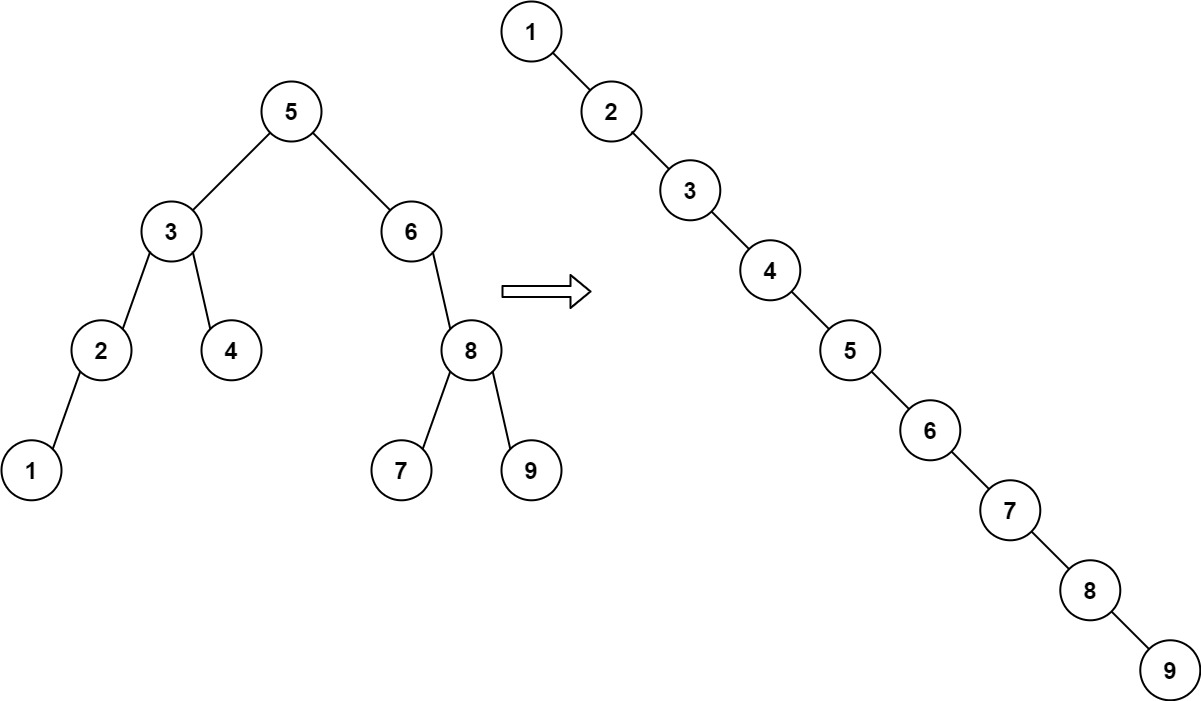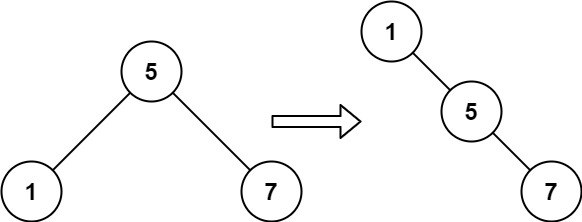# 897. Increasing Order Search Tree

## 897. Increasing Order Search Tree

Given the `root` of a binary search tree, rearrange the tree in in-order so that the leftmost node in the tree is now the root of the tree, and every node has no left child and only one right child.

Example 1:```Input: root = [5,3,6,2,4,null,8,1,null,null,null,7,9]
Output: [1,null,2,null,3,null,4,null,5,null,6,null,7,null,8,null,9]
```

Example 2:```Input: root = [5,1,7]
Output: [1,null,5,null,7]
```

Constraints:

• The number of nodes in the given tree will be in the range `[1, 100]`.
• `0 <= Node.val <= 1000`

## The solution to the above question is -

/**
* Definition for a binary tree node.
* struct TreeNode {
*     int val;
*     TreeNode *left;
*     TreeNode *right;
*     TreeNode() : val(0), left(nullptr), right(nullptr) {}
*     TreeNode(int x) : val(x), left(nullptr), right(nullptr) {}
*     TreeNode(int x, TreeNode *left, TreeNode *right) : val(x), left(left), right(right) {}
* };
*/
class Solution {
public:
TreeNode* ans=NULL;
void inorder(TreeNode* root){
if(root==NULL)return;
inorder(root->left);
root->left=NULL;
ans->right=root;
ans=root;
inorder(root->right);

}

TreeNode* increasingBST(TreeNode* root) {
if(root==NULL)return root;
TreeNode* res= new TreeNode(0);
ans=res;
inorder(root);
return res->right;
}
};